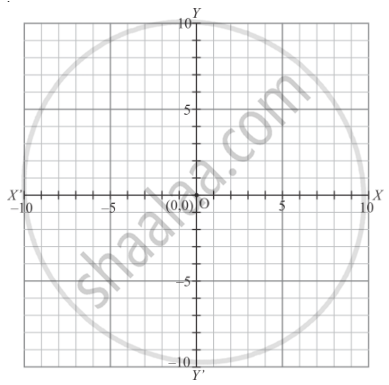# The Abscissa and Ordinate of the Origin Are - Mathematics

MCQ

The abscissa and ordinate of the origin are

• (0, 0)

• (1, 0)

• (0, 1)

• (1 , 1)

#### Solution

As we know that:

The distance of a point from y−axis is called its x−coordinate or abscissa.

The distance of a point from x−axis is called its y−coordinate or ordinate.

The coordinate axes divide the plane into four equal parts which are known as quadrants.

The point of intersection of the coordinate axes is called the origin and the coordinates of origin are (0,0)The origin is shown in the graph

Concept: Coordinate Geometry
Is there an error in this question or solution?

#### APPEARS IN

RD Sharma Mathematics for Class 9
Chapter 8 Co-ordinate Geometry
Exercise 8.2 | Q 2 | Page 7

Share